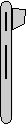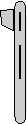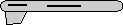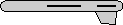# Maths - Axis-Angle to Matrix - Sample Orientations

## Sample Rotations

In order to try to explain things and give some examples we can try I thought it might help to show the rotations for a finite subset of the rotation group. We will use the set of rotations of a cube onto itself, this is a permutation group which gives 24 possible rotations as explaned on this page.

In the following table we will need to know what quadrant the results are in, so I have taken some sample results from Math.atan2

heading applied first giving 4 possible orientations:reference orientation

angle = 0 degrees
axis = 1,0,0

• c =cos(angle) = 1
• s = sin(angle) = 0
• t =1 - c = 0
[R] =
 t*x*x + c = 1 t*x*y - z*s = 0 t*x*z + y*s = 0 t*x*y + z*s = 0 t*y*y + c = 1 t*y*z - x*s = 0 t*x*z - y*s = 0 t*y*z + x*s = 0 t*z*z + c = 1
 1 0 0 0 1 0 0 0 1rotate by 90 degrees about y axis

angle = 90 degrees
axis = 0,1,0

• c =cos(angle) = 0
• s = sin(angle) = 1
• t =1 - c = 1
[R] =
 t*x*x + c = 0 t*x*y - z*s = 0 t*x*z + y*s = 1 t*x*y + z*s = 0 t*y*y + c = 1 t*y*z - x*s = 0 t*x*z - y*s = 1 t*y*z + x*s = 0 t*z*z + c = 0
 0 0 1 0 1 0 -1 0 0rotate by 180 degrees about y axis

angle = 180 degrees
axis = 0,1,0

• c =cos(angle) = -1
• s = sin(angle) = 0
• t =1 - c = 2
[R] =
 t*x*x + c = -1 t*x*y - z*s = 0 t*x*z + y*s = 0 t*x*y + z*s = 0 t*y*y + c = 1 t*y*z - x*s = 0 t*x*z - y*s = 0 t*y*z + x*s = 0 t*z*z + c = -1
 -1 0 0 0 1 0 0 0 -1rotate by 270 degrees about y axis

angle = 90 degrees
axis = 0,-1,0

or

angle = -90 degrees
axis = 0,1,0

• c =cos(angle) = 0
• s = sin(angle) = 1 or -1
• t =1 - c = 1

[R] =
 t*x*x + c = 0 t*x*y - z*s = 0 t*x*z + y*s = -1 t*x*y + z*s = 0 t*y*y + c = 1 t*y*z - x*s t*x*z - y*s = 1 t*y*z + x*s = 0 t*z*z + c = 0
 0 0 -1 0 1 0 1 0 0

Then apply attitude +90 degrees for each of the above: (note: that if we went on to apply bank to these it would just rotate between these values, the straight up and streight down orientations are known as singularities because they can be fully defined without using the bank value)angle = 90 degrees
axis = 0,0,1

• c =cos(angle) = 0
• s = sin(angle) = 1
• t =1 - c = 1
[R] =
 t*x*x + c = 0 t*x*y - z*s = -1 t*x*z + y*s =0 t*x*y + z*s = 1 t*y*y + c = 0 t*y*z - x*s t*x*z - y*s t*y*z + x*s t*z*z + c
 0 -1 0 1 0 0 0 0 1angle = 120 degrees
axis = 0.5774,0.5774,0.5774

• c =cos(angle) = 0.866
• s = sin(angle) = -0.5
• t =1 - c = 0.134
[R] =
 t*x*x + c t*x*y - z*s t*x*z + y*s t*x*y + z*s t*y*y + c t*y*z - x*s t*x*z - y*s t*y*z + x*s t*z*z + c
 0 0 1 1 0 0 0 1 0angle = 180 degrees
axis = 0.7071,0.7071,0

• c =cos(angle) = -1
• s = sin(angle) = 0
• t =1 - c = 2
[R] =
 t*x*x + c t*x*y - z*s t*x*z + y*s t*x*y + z*s t*y*y + c t*y*z - x*s t*x*z - y*s t*y*z + x*s t*z*z + c
 0 1 0 1 0 0 0 0 -1angle = 120 degrees
axis = -0.5774,-0.5774,0.5774

• c =cos(angle) = 0.866
• s = sin(angle) = -0.5
• t =1 - c = 0.134
[R] =
 t*x*x + c t*x*y - z*s t*x*z + y*s t*x*y + z*s t*y*y + c t*y*z - x*s t*x*z - y*s t*y*z + x*s t*z*z + c
 0 0 -1 1 0 0 0 -1 0

Or instead apply attitude -90 degrees (also a singularity):angle = 90 degrees
axis = 0,0,-1

(equivilant rotation to:
angle = -90 degrees
axis = 0,0,1)

• c =cos(angle) = 0
• s = sin(angle) = 1
• t =1 - c = 1
[R] =
 t*x*x + c t*x*y - z*s t*x*z + y*s t*x*y + z*s t*y*y + c t*y*z - x*s t*x*z - y*s t*y*z + x*s t*z*z + c
 0 1 0 -1 0 0 0 0 1angle = 120 degrees
axis = -0.5774,0.5774,-0.5774

• c =cos(angle) = 0.866
• s = sin(angle) = -0.5
• t =1 - c = 0.134
[R] =
 t*x*x + c t*x*y - z*s t*x*z + y*s t*x*y + z*s t*y*y + c t*y*z - x*s t*x*z - y*s t*y*z + x*s t*z*z + c
 0 0 1 -1 0 0 0 -1 0angle = 180 degrees
axis = -0.7071,0.7071,0

• c =cos(angle) = -1
• s = sin(angle) = 0
• t =1 - c = 2
[R] =
 t*x*x + c t*x*y - z*s t*x*z + y*s t*x*y + z*s t*y*y + c t*y*z - x*s t*x*z - y*s t*y*z + x*s t*z*z + c
 0 -1 0 -1 0 0 0 0 -1angle = 120 degrees
axis = 0.5774,-0.5774,-0.5774

• c =cos(angle) = 0.866
• s = sin(angle) = -0.5
• t =1 - c = 0.134
[R] =
 t*x*x + c t*x*y - z*s t*x*z + y*s t*x*y + z*s t*y*y + c t*y*z - x*s t*x*z - y*s t*y*z + x*s t*z*z + c
 0 0 -1 -1 0 0 0 1 0

Normally we dont go beond attitude + or - 90 degrees because thes are singularities, instead apply bank +90 degrees:angle = 90 degrees
axis = 1,0,0

• c =cos(angle) = 0
• s = sin(angle) = 1
• t =1 - c = 1
[R] =
 t*x*x + c t*x*y - z*s t*x*z + y*s t*x*y + z*s t*y*y + c t*y*z - x*s t*x*z - y*s t*y*z + x*s t*z*z + c

 1 0 0 0 0 -1 0 1 0angle = 120 degrees
axis = 0.5774,0.5774,-0.5774

• c =cos(angle) = 0.866
• s = sin(angle) = -0.5
• t =1 - c = 0.134
[R] =
 t*x*x + c t*x*y - z*s t*x*z + y*s t*x*y + z*s t*y*y + c t*y*z - x*s t*x*z - y*s t*y*z + x*s t*z*z + c
 0 1 0 0 0 -1 -1 0 0angle = 180 degrees
axis = 0,0.7071,-0.7071

• c =cos(angle) = -1
• s = sin(angle) = 0
• t =1 - c = 2
[R] =
 t*x*x + c t*x*y - z*s t*x*z + y*s t*x*y + z*s t*y*y + c t*y*z - x*s t*x*z - y*s t*y*z + x*s t*z*z + c
 -1 0 0 0 0 -1 0 -1 0angle = 120 degrees
axis = 0.5774,-0.5774,0.5774

• c =cos(angle) = 0.866
• s = sin(angle) = -0.5
• t =1 - c = 0.134
[R] =
 t*x*x + c t*x*y - z*s t*x*z + y*s t*x*y + z*s t*y*y + c t*y*z - x*s t*x*z - y*s t*y*z + x*s t*z*z + c
 0 -1 0 0 0 -1 1 0 0

Apply bank +180 degrees:angle = 180 degrees
axis = 1,0,0

• c =cos(angle) = -1
• s = sin(angle) = 0
• t =1 - c = 2
[R] =
 t*x*x + c t*x*y - z*s t*x*z + y*s t*x*y + z*s t*y*y + c t*y*z - x*s t*x*z - y*s t*y*z + x*s t*z*z + c
 1 0 0 0 -1 0 0 0 -1angle = 180 degrees
axis = 0.7071,0,-0.7071

• c =cos(angle) = -1
• s = sin(angle) = 0
• t =1 - c = 2
[R] =
 t*x*x + c t*x*y - z*s t*x*z + y*s t*x*y + z*s t*y*y + c t*y*z - x*s t*x*z - y*s t*y*z + x*s t*z*z + c
 0 0 -1 0 -1 0 -1 0 0angle = 180 degrees
axis = 0,0,1

• c =cos(angle) = -1
• s = sin(angle) = 0
• t =1 - c = 2
[R] =
 t*x*x + c t*x*y - z*s t*x*z + y*s t*x*y + z*s t*y*y + c t*y*z - x*s t*x*z - y*s t*y*z + x*s t*z*z + c
 -1 0 0 0 -1 0 0 0 1angle = 180 degrees
axis = 0.7071,0,0.7071

• c =cos(angle) = -1
• s = sin(angle) = 0
• t =1 - c = 2
[R] =
 t*x*x + c t*x*y - z*s t*x*z + y*s t*x*y + z*s t*y*y + c t*y*z - x*s t*x*z - y*s t*y*z + x*s t*z*z + c
 0 0 1 0 -1 0 1 0 0

Apply bank -90 degrees:angle = 90 degrees
axis = -1,0,0

(equivilant rotation to:
angle = -90 degrees
axis = 1,0,0)

• c =cos(angle) = 0
• s = sin(angle) = 1
• t =1 - c = 1
[R] =
 t*x*x + c t*x*y - z*s t*x*z + y*s t*x*y + z*s t*y*y + c t*y*z - x*s t*x*z - y*s t*y*z + x*s t*z*z + c
 1 0 0 0 0 1 0 -1 0angle = 120 degrees
axis = -0.5774,0.5774,0.5774

• c =cos(angle) = 0.866
• s = sin(angle) = -0.5
• t =1 - c = 0.134
[R] =
 t*x*x + c t*x*y - z*s t*x*z + y*s t*x*y + z*s t*y*y + c t*y*z - x*s t*x*z - y*s t*y*z + x*s t*z*z + c
 0 -1 0 0 0 1 -1 0 0angle = 180 degrees
axis = 0,0.7071,0.7071

• c =cos(angle) = -1
• s = sin(angle) = 0
• t =1 - c = 2
[R] =
 t*x*x + c t*x*y - z*s t*x*z + y*s t*x*y + z*s t*y*y + c t*y*z - x*s t*x*z - y*s t*y*z + x*s t*z*z + c
 -1 0 0 0 0 1 0 1 0angle = 120 degrees
axis = -0.5774,-0.5774,-0.5774

• c =cos(angle) = 0.866
• s = sin(angle) = -0.5
• t =1 - c = 0.134
[R] =
 t*x*x + c t*x*y - z*s t*x*z + y*s t*x*y + z*s t*y*y + c t*y*z - x*s t*x*z - y*s t*y*z + x*s t*z*z + c
 0 1 0 0 0 1 1 0 0